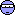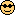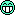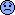I m going to give SVP another try because I hate the motion stutter on my JVC RS-25 projector. I installed everything I need and it works almost perfect exept for madVR in combination with SVP:

My projector supports 1080P23, 1080P24, 1080P50 and 1080P60. I ve set my desktop resolution in my NVIDIA control panel to 1920x1080@60 and SVP to frame rate to screens refresh rate. The problem is that I get a lot of dropped frames in madVR (one dropped frame every 2 minutes reported my madVR). I can understand this (I think) because madVR needs an exact match of the fps of the content that is playing (or a double of that I think). So without SVP i set my NVIDIA control panel to EXACTLY 1920x1080@23,976 (when running a Blu-Ray movie) and I get 1-4 dropped frames during a 2 hour movie which is great. When using SVP it of course has no sense to use it without setting my NVIDIA output to 60Hz. Will I not be able to use madVR and SVP?

While I think that one dropped frame of 2*60*60=7200 is acceptable for 99.9999% of usersyou still got two options:
1. ReClock, or
2. Check "Frame blending to screen refresh rate" option in profile

MAG79, please correct me if I'm wrongChainik
You are rightChainik
what do you mean exactly? I get one very noticable 'hickup' (dropped frame) every two minutes during a movie so that s far from ideal if you ask me.

ReClock is no option for me because I need digital output (for my D-Box system).
What does the frame blending option do exactly?It does ConvertFps().

THX-UltraII
What does the frame blending option do exactly?

It adds ConvertFps() to the end of processing chain to convert from 23.976*5/2 to the exact measured refresh rate of the screen.

BTW, I hope the projector is one and only active screen in Windows? Or at least the primary one.

Chainik wrote:

THX-UltraII
What does the frame blending option do exactly?

It adds ConvertFps() to the end of processing chain to convert from 23.976*5/2 to the exact measured refresh rate of the screen.

BTW, I hope the projector is one and only active screen in Windows? Or at least the primary one.

my projector is the primary screen.
Checking the frame blending option tops out my cpu usage to 95-98% which of course causes troubles. Do I leave the setting 'framerate change' on 'to screen refresh rate'?

THX-UltraII
Do I leave the setting 'framerate change' on 'to screen refresh rate'?
absolutely

option tops out my cpu usage to 95-98% which of course causes troubles
OK, new planOpen text file %appdata%\SVP\Ini\SVPMgr.ini and somewhere near 40th line change "maxden" to, for example, 2000 and "FPSDifference" to 0.02.
Restart SVP.

Then after opening video check the AVS script used. It should contain some big numbers in "multinum" and "multiden", not the usual 5 and 2.
And finally take a look at the file %appdata%\SVP\Ini\MediaInfo.ini:
- "FPS" - source video frame rate
- "num", "den"
- "Fres" = FPS*num/den
- "MonFreqD3D" - measured screen frame rate

Chainik
What do you mean with 'check the AVS script used'?

"show" - "avs script from last play"

yeah I know that current English translations're just awfulSample MediaInfo.ini after tuning "maxden" and "FPSDifference" values:

FileName=Camelot.S01E01.720p.HDTV.x264.Eng.mkv
FilePath=D:\video\Camelot - Season 1 [720p] (Eng+Sub)
FPS=23.976 <== source frame rate
Xish=1280
Yish=720
num=382 <== numerator
den=153 <== denominator

numOut=0
denOut=0
frameorder=
Fres=59.862 <== frame rate after SVP = input frame rate for madVR
ExAspect=6250
NewAspect=5625
CBHWidth=1280
CBHHeight=720
StrWidth=1280
StrHeight=720
CrpWidth=1280
CrpHeight=720
ResWidth=1280
ResHeight=720
MonWidth=1440
MonHeight=900
MonFreq=60 <== screen frame rate reported by Windows
MonFreqD3D=59.882 <== actual screen frame rate

OptProfile=1280x720@24

did the following in the SVPMgr.ini:

;===PROFILES===
;Nearest greater by pixelrate (1-on/0-off)
UPFindMethodProf=0

;Prpfile filter by framerate (1-on/0-off)
FPSPriority=0

;===SMOOTH FACTOR CALCULATION===
;Maximum denominator of smooth factor
maxden=2000

;Detection precision of smooth factor to screen refrech rate, Hz
FPSDifference=0.02

;smooth correction of video with variable framerate and incorrectly detected framerate by detectiong of FPS changes (0-off), when have a problems recomended value: 0.1 (fps)
deltaFPS=0.1

;Interval of parameters updating

when smooth correction is on (msec)

this is the ffdshow.avs I get:

svp_scheduler=true
SetMemoryMax(1024)
svp_cache_reduce=true
svp_flow_lib="C:\Program Files (x86)\SVP\Plugins\MVTools2\libflowgpu.dll"
multinum=5
multiden=2
algo=13
block=false
ml=0
search=4
blend=true
dist=2
pel=1
sharp=0
Recalc=0
blkh=32
blkv=32
overlap=0
overlapv=0
wid_den=1
hei_den=1

SetMTMode(3,7)
ffdShow_source()
Assert(width==1920 && height==1080,"Frame size "+string(width)+"x"+string(height)+" instead of 1920x1080")
delim=":"
WriteFileStart("C:\Users\sander\AppData\Roaming\SVP\Logs\avsFPS.txt", "FramerateNumerator()", "delim", "FramerateDenominator()")
SetMTMode(2)
src=last
wid_den==1 ? last : \
hei_den==1 ? LanczosResize(int(width/wid_den/2)*2,height) : LanczosResize(int(width/wid_den/2)*2,int(height/hei_den/2)*2)
finest=pel==1 ? super : MFinest(super)
backward_vec=MAnalyse(super, isb=true, blksize=blkh, blksizev=blkv, overlap=overlap, overlapv=overlapv, search=search, searchparam=dist, pelsearch=dist, plevel=0)
forward_vec=MAnalyse(super, isb=false, blksize=blkh, blksizev=blkv, overlap=overlap, overlapv=overlapv, search=search, searchparam=dist, pelsearch=dist, plevel=0)
backward_vec = Recalc==0 ? backward_vec : MRecalculate(super, backward_vec, blksize=blkh2, blksizev=blkv2, overlap=int(overlap/4)*2, overlapv=int(overlapv/4)*2, search=search, searchparam=dist)
forward_vec  = Recalc==0 ? forward_vec  : MRecalculate(super, forward_vec, blksize=blkh2, blksizev=blkv2, overlap=int(overlap/4)*2, overlapv=int(overlapv/4)*2, search=search, searchparam=dist)
smooth_video

distributor()

And I don t have a Mediainfo.ini file in my Ini folderTHX-UltraII
And I don t have a Mediainfo.ini file in my Ini folder

It just can't be.
Are you sure you look into "C:\Users\sander\AppData\Roaming\SVP\Ini", NOT "C:\Program Files (x86)\SVP\Ini"?
And  SVPMgr.ini should be edited there too, not in "Program files".

THX-UltraII
To avoid mistakes use SVP Manager system tray menu: Show - Hidden Settings (in Expert Interface)

It opens right SVPMgr.ini fileThxz. I will try again tonight. but what will it actually do? and do i keep the last blending checkbox enabled and set svp to 'to screen refresh rate'?

THX-UltraII
last blending checkbox enabled - nope
set svp to 'to screen refresh rate' - yep

what will it actually do?

SVP will choose more precise interpolation rate.

MediaInfo.ini now shows:

[main]
FileName=Real Steel.m2ts
FilePath=D:\Movies\D-Box\Reel Steal
FPS=23.976
Xish=1920
Yish=1080
num=368
den=147
numOut=1102896
denOut=18375
frameorder=
Fres=60.022
ExAspect=5625
NewAspect=5625
CBHWidth=1920
CBHHeight=1080
StrWidth=1920
StrHeight=1080
CrpWidth=1920
CrpHeight=1080
ResWidth=1920
ResHeight=1080
MonWidth=1920
MonHeight=1080
MonFreq=60
MonFreqD3D=60.002
OptProfile=1920x1080@24

Cool.
So frame rate after SVP is 60.022 and your screen frame rate is 60.002.
What's the number of dropped frames now?

Chainik
I still get dropped frames but I think I know the problem:
The input frame rate for madVR needs to be EXACTLY the same as the screen frame rate that my NVIDIA outputs. I get 0 (max 3!) dropped frames whne playing a BR movie (23,976 material) with NVIDIA set at 1920x1080@23,976 (custom profile).

When I want to use SVP I (of course) have to set my NVIDIA output to 1920x1080@60,000Hz. This results in 60.022 which is 'send to' madVR but NVIDIA gets 60,002. This is a difference of 0,020. This seems not much but it s too much when doing bitstreaming like me.
Is there a way to correspond these two values EXACTLY to each other maybe?

for your info, I get the following in ediaInfo.ini with the standard maxden 20 and FPSdifference 0.12 setting:

[main]
FileName=Real Steel.m2ts
FilePath=D:\Movies\D-Box\Reel Steal
FPS=23.976
Xish=1920
Yish=1080
num=5
den=2
numOut=14985
denOut=250
frameorder=
Fres=59.94
ExAspect=5625
NewAspect=5625
CBHWidth=1920
CBHHeight=1080
StrWidth=1920
StrHeight=1080
CrpWidth=1920
CrpHeight=1080
ResWidth=1920
ResHeight=1080
MonWidth=1920
MonHeight=1080
MonFreq=60
MonFreqD3D=60.002
OptProfile=1920x1080@24

THX-UltraII
Is there a way to correspond these two values EXACTLY to each other maybe?

OK, let's set "FPSDifference" to 0.001 in SVPMgr.ini

Chainik
just tried 0.00 and now Fres shows 60,001 and MonFreqD3D 60,002 so this seems almost perfect. Can 0,00 not be used and should I give 0.001 a try tonight? What does the value do exactly?

0.00 gives me almost no drops. Tried 30 minutes of playback and only got 3 drops (and where not noticable as hick-up in the picture by the way).

and what does the setting 'maxden' exactly do when changing this from 20 to 2000? Tried 0.00 with 2000 and with that setting I got the 30 minutes-3 drops result (which seems pretty good). Also tried 0.00 with the standard 20 maxden value and got a lot of (not detectable by the eye) dropped frames. So it seems indeed that increasing maxden results in less dropped frames. But how will I know if I need 200, 500, 1000, 2000 etc? And why not use eg. 25000000 to make sure there will be no dropped frames?

THX-UltraII
what does the setting 'maxden' exactly do

It's the maximum allowed denominator value in

``<resulting frame rate> = <source frame rate> * num / den``

0.00 gives me almost no drops

Good for youThere's a little problem with big numbers in "num" and "den" - you've go no original frames in output sequence which means more visible artifacts, while with 5/2 every 5th frame is original.

But we've got your point